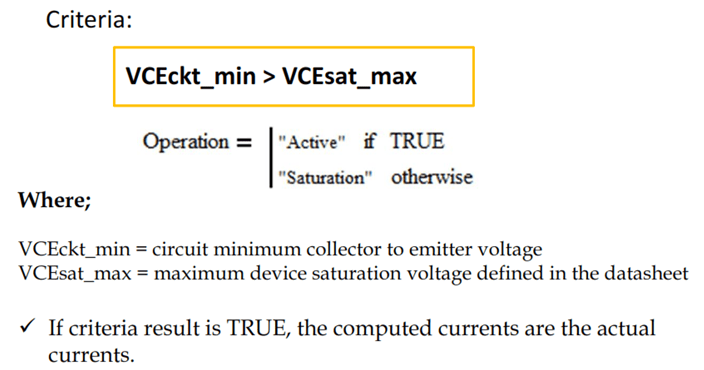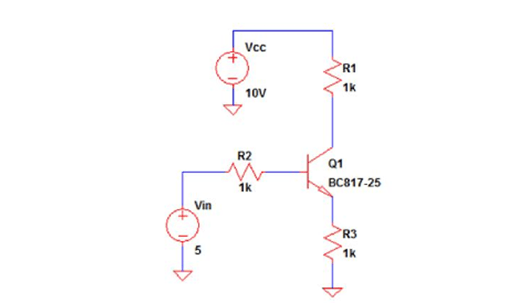# Transistor Operation Mode Determination

Transistor operation mode is can easily be determined by using mathematical equations. Don’t worry; these are not difficult equations. There are three operation modes to operate the transistor. These are cut-off, saturation and linear. In cut-off, the transistor is simply not conducting; so it is not a problem to send the transistor in this region. You can simply do that by interrupting the base current.

Saturation mode requires a bigger base current so that the transistor will stop providing current amplification. In saturation, there will be no more increase in the collector current regardless of the base current. The collector current is only limited by the collector resistance alone.

For linear mode, the base current should not that big to keep the transistor amplification property in effect. Basically, in any change in the base current there is a corresponding change in the collector current. This change is proportional to the transistor current gain or beta.

## Method #1 for Transistor Operation Mode Determination: Assume Saturation

``

The first method is to assume the circuit is already at saturation. Once the circuit is at saturation, the collector current is can be determined using the collector supply and the collector resistance. For worst case you can neglect the VCE drop of the transistor. In this transistor operation mode determination technique, once the criterion is true, the computed currents are the actual circuit currents.

Once the base current is already known, the circuit beta (βckt_max) is can be solved. A smaller base current and a higher collector current will give the worst case.

On the above method, once the criterion results is true, the transistor mode operation is definitely saturation.

## Example Transistor Operation Mode Determination using Method #1

``

In the example above, the tolerances are taken into account to get the minimum base current and maximum collector current. The computed worst circuit beta is very much lower than the minimum current gain of the transistor as specified in the datasheet, therefore no doubt that the transistor operation mode is saturation.

What we are doing in the above example is just comparing the circuit beta to the transistor minimum beta per datasheet information. Since the criterion is true, thus the computed collector current is the actual circuit current. The base current is always the actual current regardless of operation.

## Method #2 for Transistor Operation Mode Determination: Assume Linear

``

In this particular technique to know transistor operation mode, we are going to assume the circuit to be operating in the linear region. If the above criterion results to true, the transistor operation mode is definitely linear or active.

## Example Transistor Operation Mode Determination using Method #2

``

In the above example, the given VCEsat of the transistor is 0.7V. For the transistor to operate in saturation, the computed maximum VCE must be below less than 0.7V with a big margin. The resulting computed VCE is negative which means a value that is below zero and very much less than 0.7V, therefore, the transistor operation mode is definitely saturation.

## Limitations of Either Method

Method 1 is easier to use when there is no emitter resistor like our examples above. It can still be used with circuits having emitter resistors but it is complicated.

We are going to compare method 1 and 2 in below circuit with emitter resistor.

``

## Using Method #1

``
``

## Using Method #2

``

Based on above solutions, it is very evident that method #1 is difficult to use on circuits with emitter resistor. But it is very easy to use with circuits that do not have emitter resistor.